# DAV Class 6 Maths Chapter 4 Worksheet 1 Solutions

The DAV Class 6 Maths Book Solutions Pdf and DAV Class 6 Maths Chapter 4 Worksheet 1 Solutions of Ratio, Proportion and Unitary Method offer comprehensive answers to textbook questions.

## DAV Class 6 Maths Ch 4 WS 1 Solutions

Question 1.
Express the following as ratios:

(a) $$\frac{3}{5}$$
Solution:
3 : 5

(b) $$\frac{13}{17}$$
Solution:
13 : 17

(c) $$\frac{49}{36}$$
Solution:
49 : 36

(d) $$\frac{1000}{537}$$
Solution:
1000 : 537

(e) $$\frac{91}{92}$$
Solution:
91: 92

(f) $$\frac{1}{49}$$
Solution:
1 : 49

(g) 15
Solution:
15 : 1

(h) $$\frac{42}{13}$$
Solution:
42 : 13Question 2.
Find the ratio of the shaded region to that of the whole figure.

(a)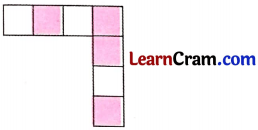Solution:
The whole figure is equally divided into 7 parts and the number of shaded parts = 4.
Hence, the required ratio = 4:7.

(b)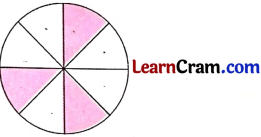Solution:
The whole figure is equally divided into 8 parts and the number of shaded parts = 3.
Hence, the required ratio = 3 : 8.Question 3.
Express the following ratios in the simplest form.

(a) 36 m to 16 m
Solution:
36 m to 16 m
== $$\frac{9}{4}$$
Hence, the simplest form of 36 m to 16 m = 9 : 4.

(b) ₹ 125 to ₹ 35
Solution:
₹ 125 to ₹ 35
=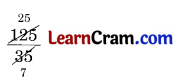= $$\frac{25}{7}$$
Hence, the required simplest form = 25 : 7.

(c) 324 : 144
Solution:
324 : 144
== $$\frac{9}{4}$$
Hence, the required simplest form = 9 : 4.

(d) 65 books to 91 books
Solution:
65 books to 91 books
=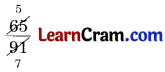= $$\frac{5}{7}$$
Hence, the required simplest form = 5 : 7.

(e) 125 : 1125
Solution:
125 : 1125
=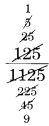= $$\frac{1}{9}$$
Hence, the required simplest form = 1 : 9.

(f) 45 hours to 36 hours
Solution:
45 hours to 36 hours
=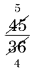= $$\frac{5}{4}$$
Hence, the required ratio = 5 : 4.Question 4.
Express the following as ratio in the simplest form.

(a) 10 cm to 10 m
Solution:
10 m = 10 × 100 = 1000 cm
∴ 10 cm to 1000 cm
== $$\frac{1}{100}$$
Hence, the required ratio = 1 : 100.

(b) 45 minutes to 4 hours
Solution:
4 hours = 4 × 60 = 240 minutes
= $$\frac{1}{2}$$
∴ 45 minutes to 240 minutes
== $$\frac{3}{16}$$
Hence, the required ratio = 3 : 16.

(c) 35 days to 3 weeks
Solution:
35 days to 3 weeks
3 weeks = 3 × 7 = 21 days
∴ 35 days to 21 days = 35 : 21
=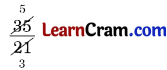= $$\frac{5}{3}$$
Hence, the required ratio = 5 : 3.

(d) 2 dozen to 1 score
Solution:
2 dozen to 1 score
2 dozen = 2 × 12 = 24 items
1 score = 20 items
∴ ratio = 24 : 20
== $$\frac{6}{5}$$
Hence, the required ratio = 6 : 5.

(e) ₹ 3.50 to 75 paise
Solution:
₹ 3.50 to 75 Paise
₹ 3.50 = 3.50 × 100 = 350 Paise
∴ ratio is 350 : 75
=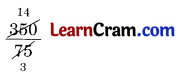= $$\frac{14}{3}$$
Hence, the required ratio = 14 : 3.

(f) 4 kg to 250 gram
Solution:
4 kg to 250 gm
4 kg = 4 × 1000 = 4000 gm
∴ ratio = 4000 : 250
=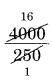= $$\frac{16}{1}$$
∴ required ratio = 16 : 1.Question 5.
Which of the following pairs of ratio is greater?

(a) 5 : 8 or 4 : 5
Solution:
5 : 8 or 4 : 5
∴ $$\frac{5}{8}$$ or $$\frac{4}{5}$$
= $$\frac{5 \times 5}{8 \times 5}$$ or $$\frac{4 \times 8}{5 \times 8}$$
= $$\frac{25}{40}$$ or $$\frac{32}{40}$$
Here, 32 > 25
∴ $$\frac{32}{40}>\frac{25}{40}$$
Hence, 4 : 5 is greater than 5 : 8.

(b) 9 : 11 or 11 : 9
Solution:
9 : 11 or 11 : 9
$$\frac{9}{11}$$ or $$\frac{11}{9}$$
= $$\frac{9 \times 9}{11 \times 9}$$ or $$\frac{11 \times 11}{9 \times 11}$$
= $$\frac{81}{99}$$ or $$\frac{121}{99}$$
Here, 81 < 121
∴ $$\frac{11}{9}>\frac{9}{11}$$
Hence, 11 : 9 is greater than 9 : 11.

(c) 23 : 24 or 15 : 16
Solution:
23 : 24 or 15 : 16
$$\frac{23}{24}$$ or $$\frac{15}{16}$$
= $$\frac{23 \times 16}{24 \times 16}$$ or $$\frac{15 \times 24}{16 \times 24}$$
= $$\frac{368}{384}$$ or $$\frac{360}{384}$$
Here, 368 > 360
∴ $$\frac{23}{24}>\frac{15}{16}$$
Hence, 23 : 24 is greater than 15 : 16.

(d) 3 : 10 or 15 : 40
Solution:
3 : 10 or 15 : 40
= $$\frac{3}{10}$$ or $$\frac{15}{40}$$
= $$\frac{3 \times 40}{10 \times 40}$$ or $$\frac{15 \times 10}{40 \times 10}$$
= $$\frac{120}{400}$$ or $$\frac{150}{400}$$
Here, 120 < 150
∴ $$\frac{15}{40}>\frac{3}{10}$$
Hence, 15 : 40 is greater than 3 : 10.Question 6.
There are 50 students in a class. If 24 of them are boys, find the ratio of boys to the girls.
Solution:
Total number of students = 50
Number of boys = 24
∴ Number of girls = 50∴24 = 26
Ratio of boys to girls = 24 : 26 = 12 : 13
Hence, the required ratio = 12 : 13.

Question 7.
Sahil ran a distance of 1.5 km and his younger sister could run only 500 m. Express the distance as ratio.
Solution:
Distance run by Sahil = 1.5 km
= 1.5 × 1000 m = 1500 m
Distance run by his sister = 500 m
∴ ratio = 1500 m : 500 m = 3 : 1
Hence, the required ratio = 3 : 1.

Question 8.
Out of 32 m long cloth, 24 metres were used for making 8 frocks. Find the ratio of:
(a) Total cloth and cloth used.
(b) Remaining cloth and cloth used.
Solution:
Total length of cloth = 32 m
Length of cloth used = 24 m
Length of remaining cloth = 32 m∴24 m = 8 m

(a) Ratio of total length to the length used = 32 : 24
= 4 : 3

(b) Ratio of remaining cloth to the used cloth = 8 : 24
= 1 : 3Question 9.
Mr. Arun earns ₹ 9500 per month and his wife earns ₹ 8000. Find the ratio of:
(a) Mr. Arun’s income to his wife’s income
(b) Wife’s income to total income
Solution:
Mr. Arun’s monthly income = ₹ 9500
His wife’s monthly income = ₹ 8000
Total income = ₹ 9500 + ₹ 8000 = ₹ 17500

(а) Ratio of Mr. Arun’s income to his wife’s income
∴ ratio = 9500 : 8000
= 95 : 80
= 19 : 16

(b) Ratio of wife’s income to total income = 8000 : 17500
= 80 : 175
= 16 : 35

Question 10.
In a dictation test of 20 words, Rohan spelled 18 words correctly. Find the ratio of:
(a) Total words to wrongly spelled words.
(b) Correctly spelled words to wrongly spelled words.
Solution:
Total number of words = 20
Number of words spelled correctly = 18
∴ Number of wrongly spelled words = 20∴18 = 2

(a) Total words to wrongly spelled words
∴ 20 : 2 = 10 : 1

(b) Correctly spelled words to wrongly spelled words
∴ 18 : 2 = 9 : 1Question 11.
Mrs. Sareen earns ₹ 2,50,000 every year and pays ₹ 24,000 as income tax. Find the ratio of:
(a) Income tax to income
(b) Income to income tax
Solution:
Total income = ₹ 2,50,000
Income tax = ₹ 24000
Ratio of income tax to income = 24,000 : 2,50,000
= 24 : 250
= 12 : 125
Ratio of income to income tax = 2,50,000 : 24,000
= 250 : 24
= 125 : 12.

Question 12.
Fill in the following blanks:

(a) Comparing two quantities by division is called _____.
Solution:
Ratio

(b) A ratio is always expressed in its _____ form.
Solution:
lowest

(c) The first term of a ratio 11 : 24 is _____ and the second term is _____.
Solution:
11, 24

(d) The ratio of the letter M in the word MATHEMATICS to the total letters in the word is _____.
Solution:
2 : 11

(e) The ratio of even numbers to odd numbers in a set of natural numbers from 1 to 25 is _____.
Solution:
12 : 13### DAV Class 6 Maths Chapter 4 Worksheet 1 Notes

Ratio:

Ratio is a comparison between two quantities. It is represented by ‘:‘
Example:
Ramesh is 10 years old and Mohan is 15 years old
∴ Ratio between their ages = $$\frac{10}{15}$$
= $$\frac{2}{3}$$ (lowest form) = 2 : 3

Ratio is always calculated between the quantities of the same unit. Therefore, ratio has no unit.
Ratio 2 : 3 may be read as ‘2 is to 3’.
Ratio is always represented in its simplest form.

Example:
Ratio 14 : 35 = 2 : 5 (simplest form).

Proportion:

Any two ratios represented is their lowest form are called proportional to each other.
Example:
Ratios 2 : 3, 4 : 6, 6 : 9 and 8 : 12 are all proportional to each other.
Symbol of proportion is : :
Ratio 6 : 9 is same as the ratio 18 : 27
So we can represent it as Product of extremes = Product of means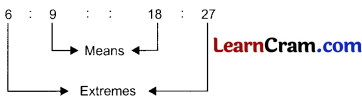6 × 27 = 9 × 18
162 = 162.Example 1:
Convert the given ratios in their simplest form:

(a) 20 : 32
Solution:
20 : 32 =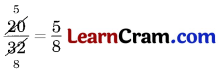Hence, the simplest form of 20 : 32 = 5 : 8.

(b) 56 : 100
Solution:
56 : 100 =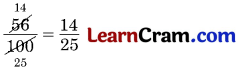Hence, the simplest form of 56 : 100 = 14 : 25.

(c) 8 : 512
Solution:
8 : 512 =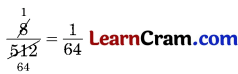Hence, the simplest form of 8 : 512 = 1 : 64.

(d) 16 : 20
Solution:
16 : 20 =Hence, the simplest form of 16 : 20 = 4 : 5.Example 2:
Find the ratio of:
(a) 20 cm to 2 m
Solution:
We know that
2 m = 2 × 100 = 200 cm
∴ 20 : 200 =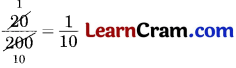Hence, the required ratio = 1 : 10.

(b) 50 grams to 2 kg
Solution:
We know that
2 kg = 2 × 1000 = 2000 g
∴ 50 : 2000 =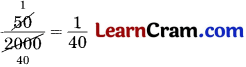Hence, the required ratio = 1 : 40.

(c) ₹ 2.50 to 2575 paise
Solution:
We know that
₹ 2.50 = 2.50 × 100 = 250 Paise
∴ 250 : 2575 =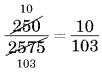Hence, the required ratio = 10 : 103.Example 3:
Check whether the following terms are in proportion.

(a) 8, 12, 10, 15
Solution:
Here, extremes are = 8 and 15
and means are = 12 and 10
Product of extremes is = 8 × 15 =120
Product of means is = 12 × 10 = 120
Product of extremes = Product of means
Hence, the given terms are in proportion and we can write 8 : 12 :: 10 : 15.

(b) 6, 8, 12, 16
Solution:
Here, extremes are = 6 and 16
and means are = 8 and 12
Product of extremes = 6 × 16 = 96
Product of means = 8 × 12 = 96
Product of extremes = Product of means
Hence, the given terms are in proportion and we can write 6 : 8 : : 12 : 16.